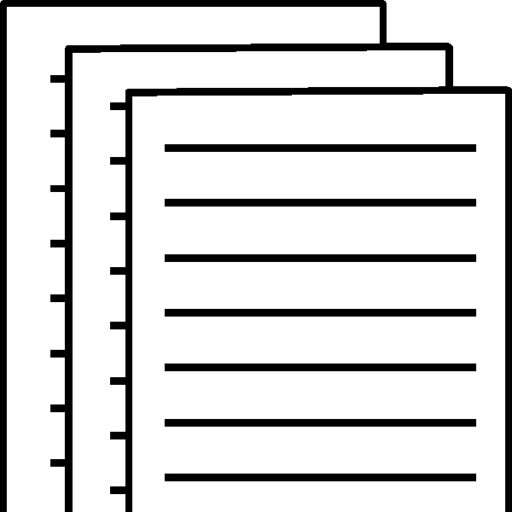## ACCT500 Week 4 Problem Set

ACCT500 – Week 4 – Problem Set

Chapter 4 (Page 86)

For the Chapter 4 problems, show all work in an Excel book, labeling each item by the problem number. Save the file in the format flastname_Unit_1_Learning_Activity.xlsx, where flastname is your first initial and your last name, and submit it to the appropriate Dropbox. For full credit, be sure to use appropriate formulas to solve each exercise or problem.

Star Inc. has Year 1 revenues of \$80 million, net income of \$9 million, assets of \$65 million, and equity of \$40 million, as well as Year 2 revenues of \$87 million, net income of \$22 million, assets of \$70 million, and equity of \$50 million. Calculate Star’s return on equity (ROE) for each year based on the DuPont method and compare it with a direct ROE measure. Next, explain why the firm’s ROE changed between Year 1 and Year 2.

Year 1 – ROE = 9,000,000 / 40,000,000 = 0.225 or 22.5%

Year 2 – ROE = 22,000,000 / 50,000,000 = 0.44 or 44%

The firms ROE changed because of increase in revenue allowing for both net income, assets, and equity to increase Due to decrease in financial leverage.

Nextime Ltd. has operating profits (EBIT) of \$87 million, a tax rate of 35%, net working capital of \$129 million, and fixed assets of \$285 million. Calculate Nextime’s return on invested capital, or ROIC. Then, describe three methods by which a firm can increase its ROIC.

ROIC = 87,000,000 x (1 – 0.35) / 129,000,000 + 285,000,000

= 56,550,000 / 414,000,000

ROIC = 0.014

Three methods by which a firm can increase it’s ROIC are; 1). Increase operating profit (EBIT), 2). Increase their net working capital, 3). By increasing fixed assets

Fixem Co. has revenue of \$125 million, property and equipment of \$42 million, and accumulated depreciation and amortization of \$6 million. Estimate the fixed asset turnover ratio.

Fixed asset turnover = 125,000,000 / 42,000,000 = 2.97

Fixed asset turnover = 125,000,000 / (42,000,000 – 6,000,000) = 3.5

Wally Wholesale has revenue of \$487,000, end-of-year receivables of \$112,000, account payables of \$70,000, and inventory of \$91,000. Assume purchases equal cost of sales of \$372,000. Estimate Wally Wholesale’s age of inventory, age of receivables, and age of payables.

Age of inventory = 91,000 / 372,000 = 0.2489.3 days

Age of receivables = 112,000 / 372,000 = 0.383.9 days

Age of payables = 70,000 / 372,000 = 0.18868.7 days

Quick-E Inc.’s current assets consist of cash of \$5 million, account receivables of \$27 million, inventory of \$37 million, and it has current liabilities of \$48 million. Calculate Quick-E’s current ratio and quick ratio.

Current ration = 5,000,000 / 48,000,000 = 0.11.4

Quick ration = 5,000,000 + 27,000,000 / 48,000,000 = 0.66

Deb Co. has interest-bearing debt of \$122 million, non–interest-bearing debt of \$33 million, and equity of \$76 million. Calculate Deb Co.’s debt-to-assets, debt-to-equity, and long-term debt-to-capital ratios.

Debt-to-assets = 155,000,000 / 76,000,000 = 2.00.671

Debt-to-equity = 122,000,000 / 76,000,000 = 1.62.04

Long-term debt-to-capital = 122,000,000 – 33,000,000 / 122,000,000 – 33,000,000 + 76,000,0000.616

89,000,000 / 89,000,000 + 76,000,000 = 1 + 76,000,000 = 77,000,000

IOU Inc. has EBIT of \$58,000, depreciation and amortization of \$12,000, interest expenses of \$21,000, principal repayments of \$17,000, and a tax rate of 35%. Calculate IOU Inc.’s interest coverage ratio and debt service coverage ratio.

Interest coverage = 58,000 / 21,000 = 2.76

Debt service = 58,000 + 0.35 + 12,000 / 21,000 (17,000 / 1 – 0.35)

= 70,000.35 / 21,000 x 26,153.85

= 70,000.35 / 549,230,850 = 0.011.5

Foerster, Stephen. Financial Management: Concepts and Applications. Prentice Hall, 01/2014. VitalBook file.

To view and download a complete answer, scroll down to the bottom to pay